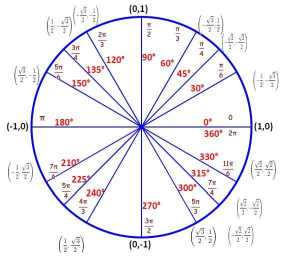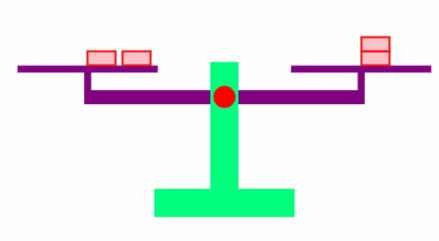AM
Animated Mathematics# Notes for IB Mathematics AA SL and HL

### Number and Algebra

Arithmetic and Geometric series, logarithms, Binomial Principles, counting principles, complex numbers, Euler form, De Moivre's theorem, powers and roots of complex numbers.

### Functions

Function concepts, inverse functions, graphs of functions, composite functions, quadratic functions, exponential and logarithmic functions, inequalities involving absolute values, graphical transformation, polynomial functions

### Statistics and Probability

Population and Data, Presentation of data, central tendency, linear correlation, introduction to probability, Venn Diagrams, combined events, independent events, Bayes theorem, random variables, Binomial and Normal Distributions,